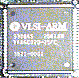# MultiplicationinstructionsThese two instructions are different from the normal arithmetical instructions in that there are restrictions on the operands, namely:

1. All operands, and the destination, must be given as simple registers.
2. You cannot use immediate values or shifted registers for operand two.
3. The destination and operand one must be different registers.
4. Lastly, you cannot specify R15 as the destination.

## MLA : Multiplication with Accumulate

```  MLA<suffix>  <dest>, <op 1>, <op 2>, <op 3>

dest = (op_1 * op_2) + op_3
```
`MLA` behaved that same as `MUL`, except that the value of operand three is added to the result. This is useful for running totals.

## MUL : Multiplication

```  MUL<suffix>  <dest>, <op 1>, <op 2>

dest = op_1 * op_2
```
`MUL` provides 32 bit integer multiplication. If the operands are signed, it can be assumed that the result is also signed.
Here is an example of multiplication:
```REM Multiplication example
REM
REM by Richard Murray 26th April 1999
REM

DIM code% 12

P%=code%
[  OPT 2
MUL     R2, R0, R1
MOV     R0, R2
MOV     PC, R14
]

REPEAT
INPUT "Number 1 : "A%
INPUT "Number 2 : "B%
PRINT "Result   : "+STR\$(USR(code%))'
UNTIL A% = 0

END
```